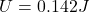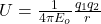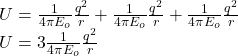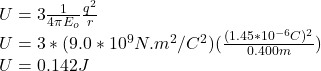## Three equal point charges, each with charge 1.45 μC , are placed at the vertices of an equilateral triangle whose sides are of length 0.400

Question

Three equal point charges, each with charge 1.45 μC , are placed at the vertices of an equilateral triangle whose sides are of length 0.400 m . What is the electric potential energy U of the system? (Take as zero the potential energy of the three charges when they are infinitely far apart.)

in progress 0
2 weeks 2021-09-02T05:56:41+00:00 1 Answers 0 views 0Explanation:

The electrostatic potential energy for a pair of charge is given by:Hence for a system of three charges the electrostatic potential energy can be found by adding up the potential energy for all possible pairs of charges.For three equal charges on the corner of an equilateral triangle,the electrostatic energy given by:Substitute the values as q=1.45μC and r=0.400m

So